# AP Board 9th Class Maths Solutions Chapter 7 Triangles Ex 7.3

AP State Syllabus AP Board 9th Class Maths Solutions Chapter 7 Triangles Ex 7.3 Textbook Questions and Answers.

## AP State Syllabus 9th Class Maths Solutions 7th Lesson Triangles Exercise 7.3Question 1.
AD is an altitude of an isosceles triangle ABC in which AB = AC. Show that, (i) AD bisects BC (ii) AD bisects ∠A.
Solution: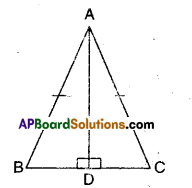Given that in ΔABC, AB = AC
i) Now in ΔABD and ΔACD
AB = AC (given)
∴ ΔABD ≅ ΔACD (∵ RHS congruence)
⇒ BD = CD (CPCT)

(CPCT of ΔABD ≅ ΔACD )Question 2.
Two sides AB, BC and median AM of one triangle ABC are respectively equal to sides PQ and QR and median PN of ΔPQR (see figure). Show that:
(i) ΔABM ≅ ΔPQN
ii) ΔABC ≅ ΔPQR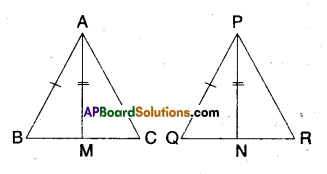Solution:
Given that
AB = PQ
AM = PN
i) Now in ΔABM and ΔPQN
AB = PQ (given)
AM = PN (given)
BM = QN (∵ BC = QR ⇒ $$\frac { 1 }{ 2 }$$BC = $$\frac { 1 }{ 2 }$$QR ⇒ BM = QN)
∴ ΔABM ≅ ΔPQN
(∵ SSS congruence)

ii) In ΔABC and ΔPQR
AB = PQ (given)
BC = QR (given)
∠ABC = ∠PQN [∵ CPCT of ΔABM and ΔPQN from (i)]
∴ ΔABC ≅ ΔPQR
(∵ SAS congruence)Question 3.
BE and CF are two equal altitudes of a triangle ABC. Using RHS congruence rule, prove that the triangle ABC is isosceles.
Solution: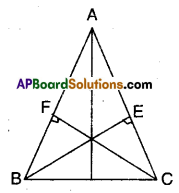In ΔABC altitude BE and CF are equal.
Now in ΔBCE and ΔCBF
∠BEC = ∠CFB (∵ given 90°)
BC = BC (common; hypotenuse)
CF = BE (given)
∴ ΔBEC ≅ ΔCBF
⇒ ∠EBC = ∠FCB (∵ CPCT)
But these are also the interior angles opposite to sides AC and AB of ΔABC.
⇒ AC = AB
Hence proved.Question 4.
ΔABC is an isosceles triangle in which AB = AC. Show that ∠B = ∠C.
(Hint : Draw AP ⊥ BQ (Using RHS congruence rule)
Solution: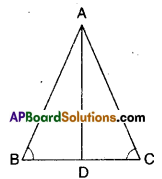Given the ΔABC is an isosceles triangle and AB = AC
Let D be the mid point of BC; Join A, D.
Now in ΔABD and ΔACD
AB = AC (given)
BD = DC (construction)
∴ ΔABD ≅ ΔACD (∵ SSS congruence)
⇒ ∠B = ∠C [∵ CPCT]

Question 5.
ΔABC is an isosceles triangle in which AB = AC. Side BA is produced to D such that AD = AB (see figure). Show that ∠BCD is a right angle.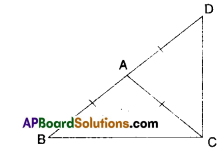Solution: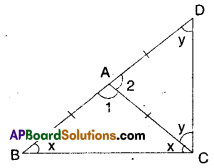Given that in ΔDBC; AB = AC; AD = AB
In ΔABC
∠ABC + ∠ACB = ∠DAC …………… (1)
[∵ exterior angle]
In ΔACD
∠ADC + ∠ACD = ∠BAC ………………(2)
∠DAC + ∠BAC = 2 ∠ACB + 2∠ACD
[∵ ∠ABC = ∠ACB
180° = 2 [∠ACB + ∠ACD]
180° = 2[∠BCD]
∴ ∠BCD = $$\frac{180^{\circ}}{2}$$ = 90°
(or)
From the figure
∠2 = x + x = 2x
∠1 = y + y = 2y
∠1 + ∠2 = 2x + 2y
180° = 2 = (x + y)
∴ x + y  = $$\frac{180^{\circ}}{2}$$ = 90°
Hence proved.Question 6.
ABC is a right angled triangle in which ∠A = 90° and AB = AC, Show that ∠B = ∠C.
Solution: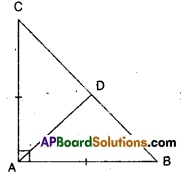Given ΔABC; AB – AC
Join the mid point D of BC to A.
AC = AB (giyen)
DC = DB (construction)
⇒ ∠C = ∠B (CPCT)Question 7.
Show that the angles of an equilateral triangle are 60° each.
Solution: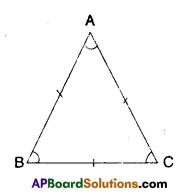Given ΔABC is an equilateral triangle
AB = BC = CA
∠A = ∠B (∵ angles opposite to equal sides)
∠B = ∠C (∵ angles opposite to equal sides)
⇒ ∠A = ∠B = ∠C = x say
Also ∠A+∠B + ∠C =180°
⇒ x + x + x = 180°
3x = 180°
⇒ x = $$\frac{180}{3}$$ = 60°
Hence proved.